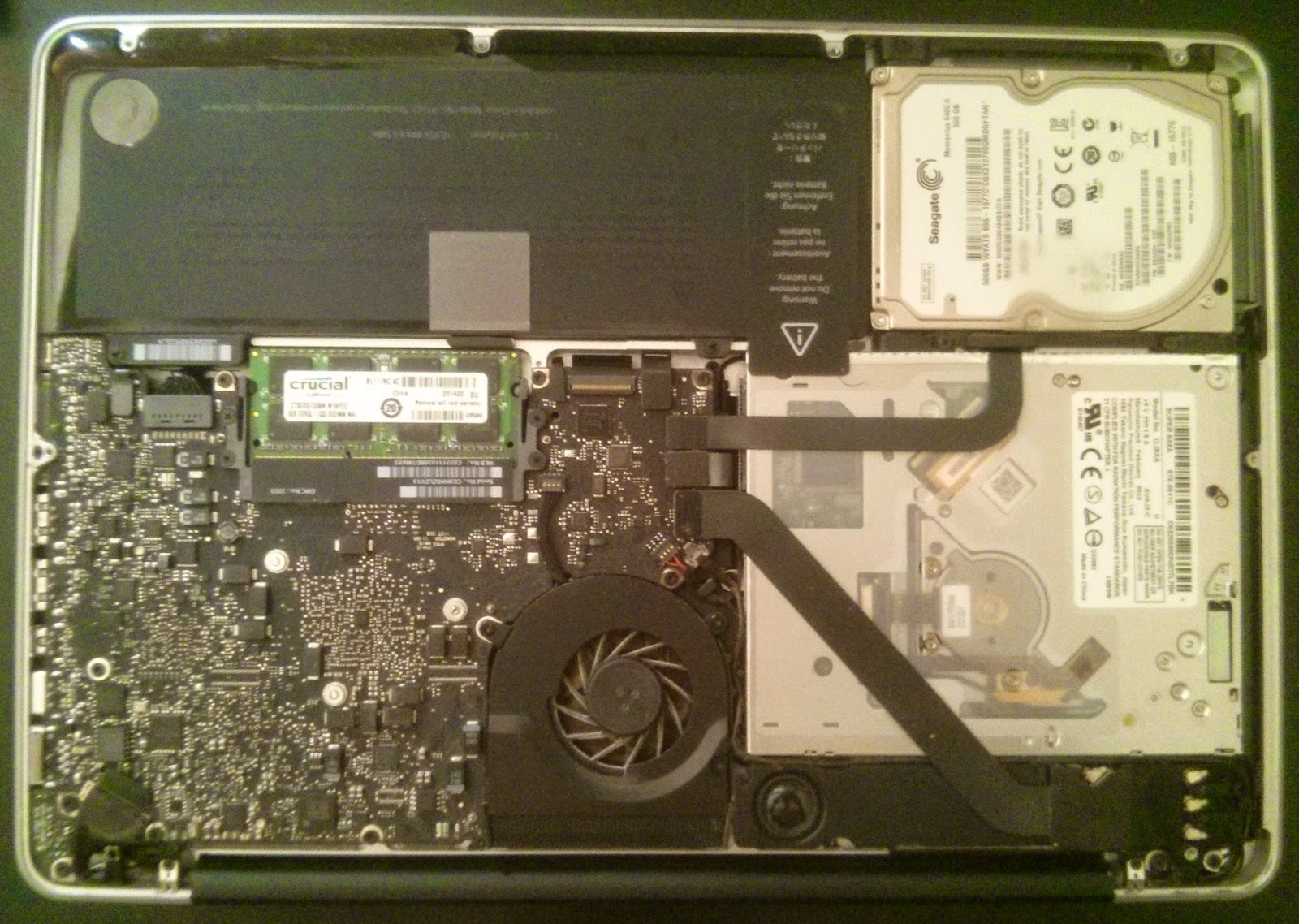## Thursday, 24 July 2014

### Paper summary : Authorization-Transparent Access Control for XML under the Non-Truman Model by Kanza et. Al., EDBT '06

• Truman model - invalid queries are modified.
• Non-truman model - invalid queries rejected.
• Security policies specified using XPath. e.g., for /Dept[Name=CS]/Course exclude //Grade
• Not only XML elements but edges and paths can also be concealed.
• Query is locally valid for dataset $D$ and set of rules $R$ if it conceals all relationships of $R$. Query is globally valid for any $D$ which conforms to some schema $S$ if $R$ is concealed for all $D$.
• Document model $D = (X, E_{D}, root_{D}, labelOf_{D}, valueOf_{D})$.$X$ refers to nodes (nodes contain metadata like ids), $E_{D}$ refers to elements of the XML (i.e., attribute values, this is, in reality, a metadata of a node). $root_{D}$ is the root node, $labelOf_{D}$ is a function $f: X \to E_{D}$ and $valueOf_{D}$ is a function $f: X \to A$ where $A$ is a set of element values of atomic nodes (nodes with no outgoing edges).
• k-concealment of relationship $(A,B)$ for some elements $A, B$ in $D$: given $b \in B$, we want a subset $A_{k} \subset A$ st (i) no element in $A_{k}$ is ancestor of another (ii) user cannot infer which among $k$ is an ancestor of $B$.
• Expansion of $D$ is $D''$, created by replacing $E_{D}$ with $E'$ (children) and $E''_{D}$ (descendents).
• A transitive closure $\bar{D}$ is an expansion of $D$ st there is an edge bet. every 2 nodes connected by direct path in $D$ and an edge from every node to itself.
• An XPath query is equivalent to evaluating the query over the transitive closure of the document.
• To prune a document expansion is to remove from $D''$ all edges that connect restricted nodes.
• Universe of expansions of $D$ is the set of all $D''$ st $prune(\bar{D}$) = prune($D'')$. Denote this by $U_{R}(D)$.
• (Local validity) Query $Q$ is locally valid if $Q(D) = Q(D''), \forall D'' \in U_{R}(D)$.
• (Pseudo validity) Query $Q$ is pseudo valid if $Q(D) = Q(prune(\bar{D}))$. You can leak relationships here e.g., singleton source disclosure (example 11).
• (Global validity) $Q$ is globally valid for $R$ give schema $S$ if $Q$ is locally valid $\forall D$ that conform to $S$. This is more restrictive than local validity but offers benefits (no need to check $Q$ over all $D$ if $Q$ is globally valid, saving computation).
• $R$ is coherent if it is impossible to infer any hidden relationships from the relationships not pruned by the rules.
• $R$ has incomplete concealment in $D$ if either (i) $D$ has 3 elements $e1,e2,e3$ st $prune(\bar{D})$ has an edge from $e1$ to $e2$ and $e2$ to $e3$ but none from $e1$ to $e3$ or (ii) $D$ has 3 elements $e1,e2,e3$ st $prune(\bar{D})$ has an edge from $e1$ to $e3$ and $e2$ to $e3$ but none from $e1$ to $e2$.
• (Coherent) R is coherent if incomplete concealment does not occur in $D$.
• Encapsulating edge in $\bar{D}$: $(e1, e2)$ encapsulates $(e1' e2')$ if there is a path running through all of these edges and $e1$ appears before (or at the same spot) as $e1'$ or if $e2$ appears after (or at the same spot) as $e2'$.
• If $R$ is coherent, $\forall (e1,e2)$ in $\bar{D}$ which are removed by pruning, if there is an edge $(e1',e2')$ which is encapsulated by $(e1,e2)$, then $(e1',e2')$ should be removed too.
• Weakness of approach so far is singleton source disclosure i.e., $\exists \rho=(x1,x2) \in R$ st $(x1(D), x1x2(D))$ is not 2-concealed ($x1(D)$ means that $x1$ was the query whose answer exsits in $D$). Solution : algorithm $computeK$ is provided.
• (Theorem 1) Algo $computeK$ returns $k$ st (i) all the relationships in $R$ are k-concealed (ii) $\exists r \in R$ which is not $k+1$ concealed.

### Crucial 16GB RAM upgrade for my Macbook Pro (Late 2011)

It had become really taxing for me to work on simultaneous applications on my 4GB macbook pro. Eclipse especially consumes an inordinate amount on memory. Hence I decided to purchase a 16GB RAM card on Amazon.The innards of my Macbook Pro (late 2011)!
The difference between 4GB and 16GB is truly astounding. Now I can actually open up RStudio and Eclipse simultaneously. What a luxury :|

As an aside, it is ridiculous how shipping a RAM card from the US to Canada even with the shipping charges saves you a significant amount compared to buying the RAM card here. This is true for almost all products. Why Canada, WHY?!